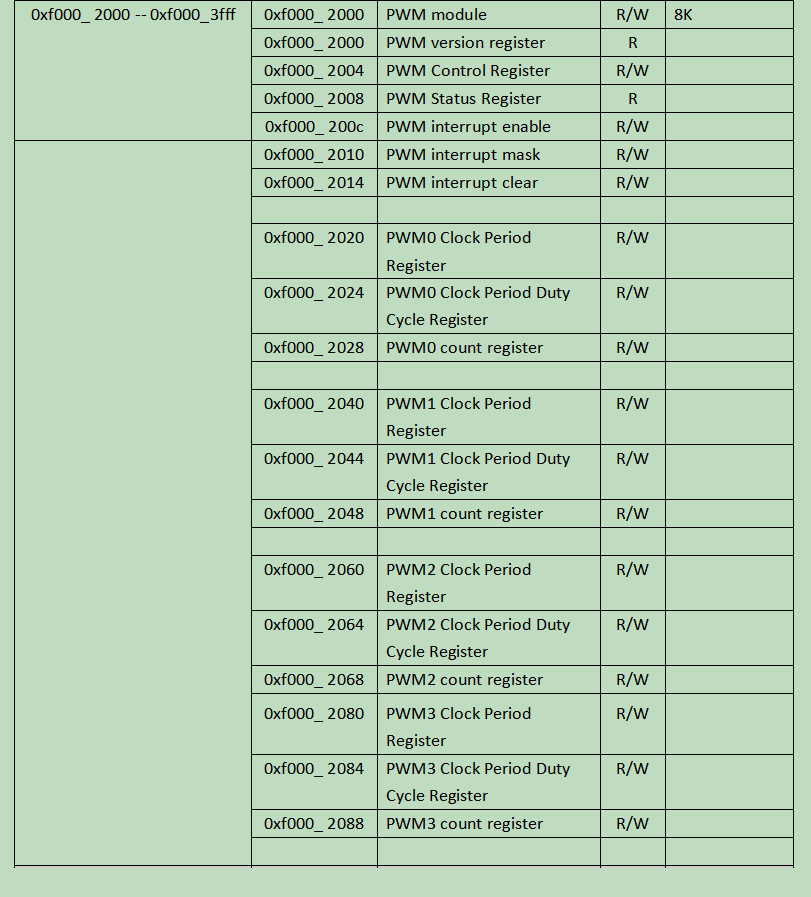# 1. Introduction to PWM

Related reference articles:

# RISC-V teaching plan

PWM (pulse-width modulation), pulse width modulation is a method of cutting the electrical signal into discrete parts to effectively reduce the average power transmitted by the electrical signal. In simple terms, PWM controls the average value of voltage and current by controlling how long the switch is open relative to when the switch is closed. The period of PWM refers to the time required to produce a full-wave. The duty cycle describes the ratio of the “on” time to the cycle, 100% is always on, 50% is half time on, and half time off (see Figure 1 for a digital signal at different duty cycles) than the waveform below).Figure 1 Waveforms of digital signals under different duty cycles

The application of PWM is very wide, because it can control the switch and set the corresponding duty cycle, it can be used as the modulation of the boost converter to convert the low voltage into a high voltage; or as an inverter circuit (inverter) circuit) to convert DC voltage to AC voltage. In fact, many digital controls are often used in conjunction with it. PWM is also used in some communication systems where duty cycles pass messages through the communication channel.

# 2. PWM design

• PWM can be set to generate a precise interrupt that approximates a timer. After the interrupt is generated, it is also processed by the PLIC.
• Each generated PWM waveform is described by a 32-bit wide register, including period, duty cycle, etc.
• PWM can be connected to GPIO output pins to generate different waveforms.
• The integer register Period Duty Cycle (PWM clock cycle duty cycle register) and Period (PWM clock cycle register) are used together to represent the real duty cycle, Duty Cycle = (Period Duty Cycle/ Period) X 100%

Figure 3 is an example with period=8 (4’b1000):Figure 2 Clock Period Register and Clock Period Duty Cycle RegisterFigure 3 Configuring the duty cycle register

• The PWM control register is a global enable for PWM, and starts to generate waveforms after it is turned on, as shown in Figure 4.Figure 4 PWM Control Register

• The PWM count register indicates how many cycles the PWM lasts. For example, if the count register is equal to 0x8000, when 0x8000 full waves have been generated, the PWM stops working. The special case is that when the counter is 0 and the enable is standing up, the PWM has been generating waveforms. The definition of the pwm count register is shown in Figure 5.Figure 5 PWM count register

• Interrupt enable, interrupt mask, and interrupt clear work together to handle interrupts, as shown in Figure 6.Figure 6 PWM interrupt related registers

• The address range where the PWM register is located is shown in Figure 7.Figure 7 The address range where the PWM register is located

# 3. PWM CPU module design (part)

FII RISC-V V2.02 and later CPUs have a bus structure, so after completing and verifying the PWM module function, you need to connect the PWM module to the bus, as shown in Figure 8, and you need to pay attention to adding PWM The base address parameter, as shown in Figure 9.Figure 8 Add chip select for PWM in busAn example of a module that generates a PWM waveform is shown in Figure 10. Send the generated interrupt request of the PWM module to the PLIC module, as shown in Figure 11.Figure 10 PWM module interface designFigure 11 Interrupt request generated by PWM module